# I Think Faster Than Light Travel is Possible. Here's Why.

Last updated: May 7, 2023

The idea that the speed of light is a limit comes from Albert Einstein's theory of Special Relativity.

The theory says that the speed of light is the only speed that's the same for all observers, and it's the same in a vacuum.

This article debunks a common misconception by showing how particles can move at the speed of light without infinite energy.

The Higgs field and its condensed form are Lorentz-scalar invariant under Poincaré transformations.

Physicists often bring up the idea that traveling faster than the speed of light can cause time-travel paradoxes, which is why faster than light travel is important.

• The speed of light is the only speed that's the same for all observers, making it the limit of the speed of information.
• Misconceptions about the speed of light being a limit stem from Einstein's theory of Special Relativity.
• Particles can travel at the speed of light without infinite energy if the Higgs field is condensed and they become massless.
• The Higgs field slows down particles that have mass and its condensed form is Lorentz-scalar invariant.
• Traveling faster than the speed of light can cause time-travel paradoxes.
• Physicists have noticed time order reversal in relation to faster-than-light travel.
• There are problems with the idea of faster-than-light travel, including the causality paradox and the fact that our current theory of space-time doesn't work with quantum theory.
• We need a theory of quantum gravity to understand the possibilities of faster-than-light travel.
• It's currently implausible that any argument about faster-than-light travel would survive in a theory of quantum gravity.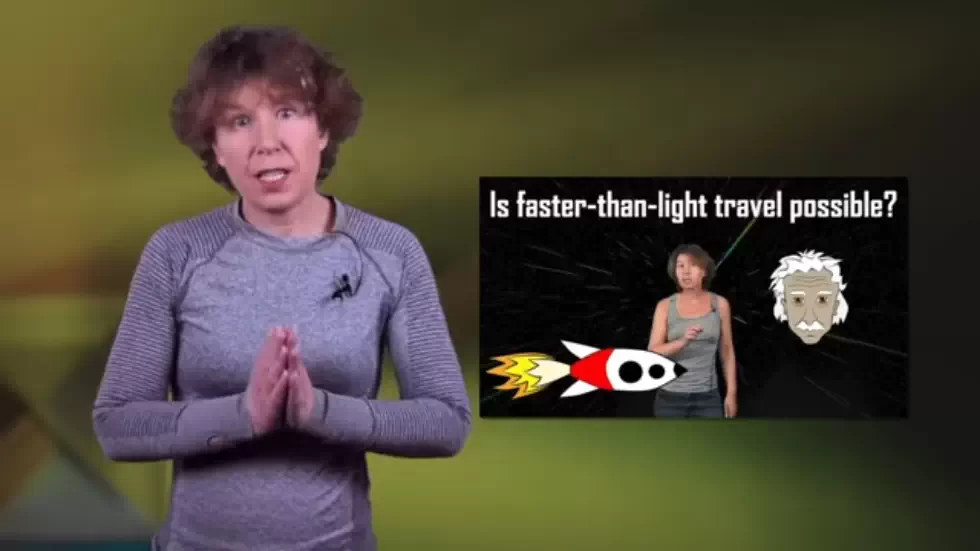## The Limit of the Speed of Light 00:00

• Intelligent life exists on other planets, but they haven't contacted us because we haven't figured out how to send information faster than light.
• The idea that the speed of light is a limit comes from Albert Einstein's theory Special Relativity.
• The speed of light is the only speed that's the same for all observers, and it's the same in vacuum.
• Light moves away from an object with a speed of light, no matter how fast the object is moving.
• It's impossible to catch up with light, and it has a consequence that you can't move faster than the speed of light.
• The energy needed to accelerate an object to the speed of light is infinite, which means that only massless objects can move at the speed of light.
• The speed of light plays a special role in Einstein's theory because it's the only speed that's the same for all observers, making it the limit of the speed of information.## Misconceptions About the Limit of the Speed of Light 00:38

• The idea that the speed of light is a limit doesn't mean that faster than light travel is forbidden in Einstein's theory.
• The theory is entirely compatible with faster-than-light travel.
• The problem seems to be that you can't accelerate from below the speed of light to above the speed of light.
• The idea that infinite energy is needed to reach the speed of light is suspect because we have a counterexample that proves otherwise.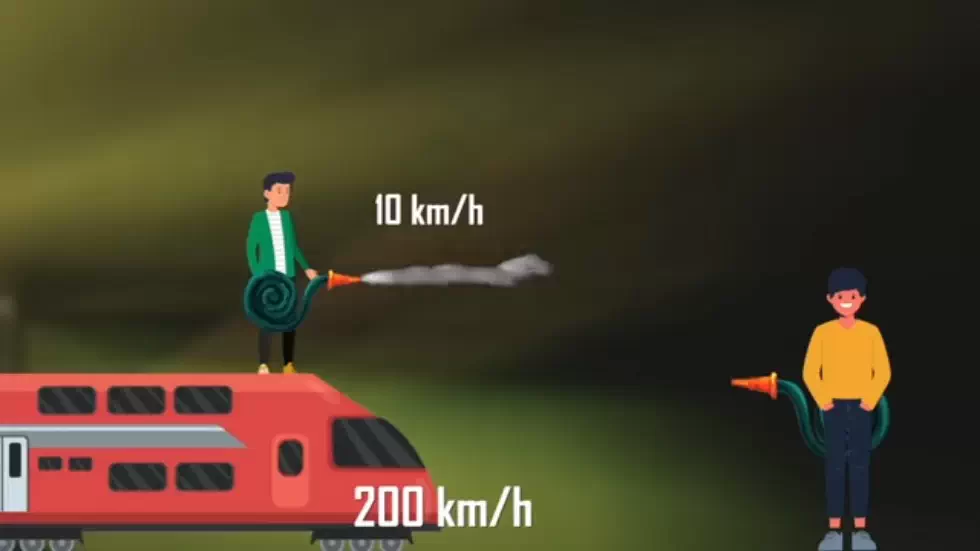## Counterexample to the Claim that Infinite Energy is Needed 07:12

• To understand the counterexample, you need to know where mass comes from.
• Mass comes from the Higgs field, which is a field that permeates all of space and gives particles their mass.
• The Higgs field is like molasses, and particles moving through it experience resistance, which is why they have mass.
• But if the Higgs field had a different value, particles could move through it without experiencing resistance, and they would be massless.
• In this case, they would also move at the speed of light, which means that massless particles don't need infinite energy to move at the speed of light.
• Therefore, the claim that infinite energy is needed to reach the speed of light is incorrect.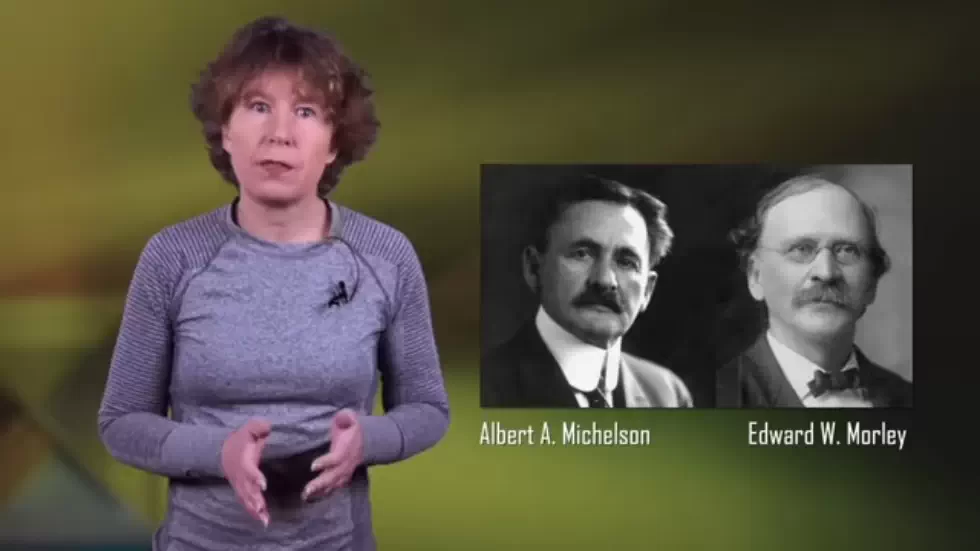## The nature of mass 07:18

• Most of the mass in objects around us is not actually mass, but rather binding energy.
• Almost all of the mass of an atom is in its nucleus, which is made up of neutrons and protons.
• Neutrons and protons are each made up of three quarks, which do have mass, but when added together, the sum is far less than the mass of the neutron or proton.
• Most of the mass of neutrons and protons comes from the strong nuclear force that holds them together, which we interpret as mass because of E=mc².
• Microscopically, most of an object is not mass, and most of a person is made up of pure energy.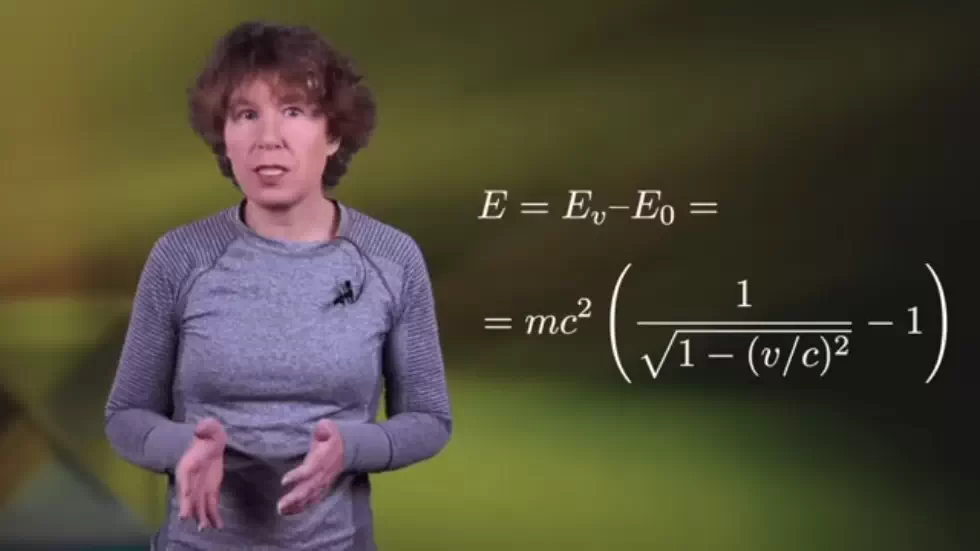## The source of mass 08:29

• Electrons and quarks do have masses, but they are very small.
• These masses come from the Higgs field, which is a field that fills the entire universe and drags on particles.
• The Higgs field is not to be confused with the Higgs boson.
• The Higgs field's condensed form is what gives fundamental particles mass.
• In the early universe, particles did not have mass because the Higgs field was not condensed.
• The Higgs field condensed when the universe cooled, and this phase transition called “electroweak symmetry breaking” happened about 10^-11 seconds after the Big Bang at a temperature of 10^15 Kelvin.
• The energy released in this phase transition was finite, which is important because if it hadn't been, we wouldn't be here.## The Higgs field and the speed of light 08:58

• The Higgs field slows down particles that have mass by sticking to them.
• Photons are massless, which means they don't feel the Higgs field at all.
• The Higgs-condensate looks the same for everyone, regardless of how fast they move, which is not the case for the aether.
• The Higgs field and its condensed form are Lorentz-scalar invariant under Poincare transformations.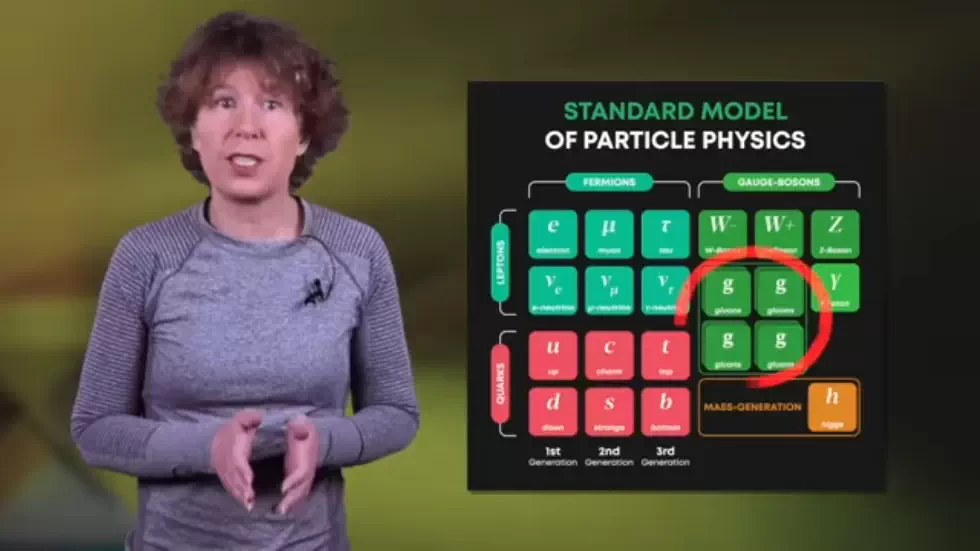## The speed of light barrier 12:22

• We can't just uncondense the Higgs field to travel at the speed of light because it would evaporate the traveler.
• Mathematically, if the factor of the equation goes to zero but the mass also goes, then the ratio can remain finite.
• This doesn't help us travel faster than the speed of light.

• Physicists often bring up the idea that traveling faster than the speed of light can cause time-travel paradoxes.
• The argument is that if Alice observes a spaceship going faster than the speed of light, then Bob, who is moving relative to Alice, would see the spaceship going back in time.
• This is because Bob sees different events happening simultaneously due to his relative motion.

## Time Order Reversal for Faster Than Light Travel 14:40

• In special relativity, events that happen at equal times are on straight lines for Bob, not on horizontal lines.
• Bob sees the order of events as egg first smashing to the ground and then gets dropped.
• For Bob, the time order of faster than light ship is reversed.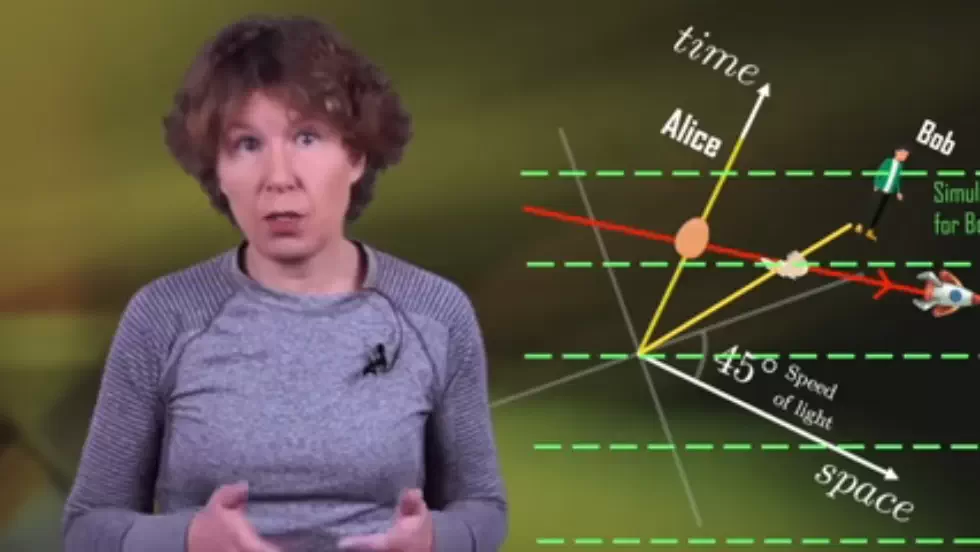## Why Bob's Observation Matters 15:03

• You may argue that it doesn't matter what Bob sees, but physicists have noticed this time order reversal.
• Imagine that there is a faster-than-light spaceship that can go back in time according to Bob.
• If the time on spaceship goes forward in this way, then you can give a message to the guys as they come by.

• The time travel argument is correct in special relativity, but it is not in general relativity.
• Imagine that there is a spaceship that can go faster than light and according to you, it goes back in time.
• If you send a message to your younger self not to watch this video, then you'd never have sent the message in the first place, so did you or didn't you watch it?
• This type of construction is also called a time-like closed loop, and it's a loop in time.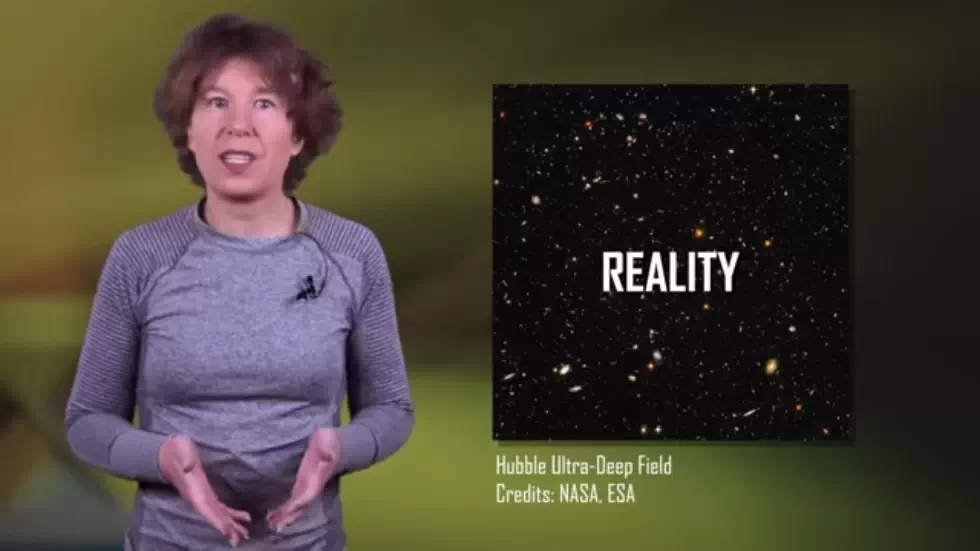## The Co-Moving Frame 18:51

• The real universe contains stuff, and you can calculate the average velocity that it moves with.
• You can define absolute rest to be motion that has no relative velocity to the average of all that stuff.
• It's called the co-moving frame, which is the reference frame that moves along with matter in the universe.
• In a universe with general relativity, if you are moving the stuff, you are in the co-moving frame.

## The Possibility of Faster Than Light Travel 20:47

• Faster-than-light travel does not necessarily imply time-travel paradoxes.
• We know that our current theory of space-time, General Relativity, can't be correct because it doesn't work together with quantum theory.
• We need a theory of quantum gravity, and we still don't have one.
• This is why it's extremely implausible that any argument about faster-than-light travel would survive in the to-be-found theory of quantum gravity.

## Importance of Active Learning 22:11

• The speaker believes that everyone can understand Einstein's theories today.
• However, passive learning through watching YouTube videos is not enough.
• Active engagement with the material is necessary to fully comprehend the theories.
• The sponsor Brilliant offers courses on science and mathematics to help with active learning.